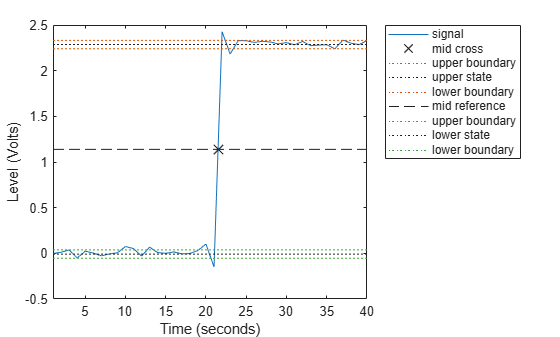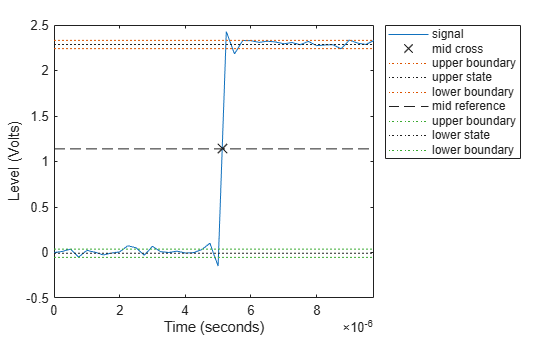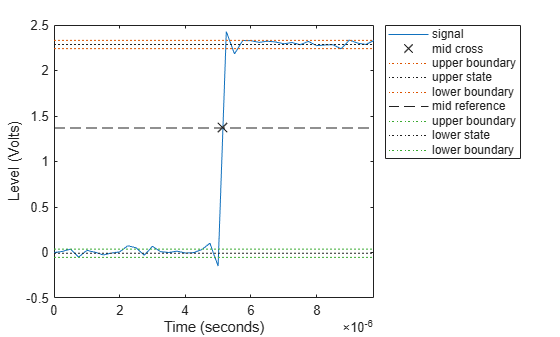# midcross

Mid-reference level crossing for bilevel waveform

## Syntax

C = midcross(X) C = midcross(X,FS) C = midcross(X,T) [C,MIDLEV] = midcross(...) C = midcross(X,Name,Value) midcross(...) 

## Description

C = midcross(X) returns a vector, C, of time instants where each transition of the input signal, X, crosses the 50% reference level. The sample instants correspond to the indices of the input vector. Because midcross uses interpolation to determine the crossing instant, C may contain values that do not correspond to sampling instants. To determine the transitions, midcross estimates the state levels of X by a histogram method. midcross identifies all intervals which cross the upper-state boundary of the low state and the lower-state boundary of the high state. The low-state and high-state boundaries are expressed as the state level plus or minus a multiple of the difference between the state levels. See State-Level Tolerances.

C = midcross(X,FS) specifies the sample rate, FS, in hertz as a positive scalar. The first sample instant corresponds to t=0. Because midcross uses interpolation to determine the crossing instant, C may contain values that do not correspond to sampling instants.

C = midcross(X,T) specifies the sample instants, T, as a vector with the same number of elements as X. Because midcross uses interpolation to determine the crossing instant, C may contain values that do not correspond to sampling instants.

[C,MIDLEV] = midcross(...) returns the waveform value corresponding to the mid-reference level.

C = midcross(X,Name,Value) returns the time instants corresponding to mid-reference level crossings with additional options specified by one or more Name,Value pair arguments.

midcross(...) plots the signal and marks the location of the mid-crossings (mid-reference level instants) and the associated reference levels. midcross also plots the state levels with upper and lower state boundaries.

## Input Arguments

 X Bilevel waveform. X is a real-valued row or column vector. FS Sample rate in hertz. T Vector of sample instants. The length of T must equal the length of the bilevel waveform, X.

### Name-Value Arguments

Specify optional comma-separated pairs of Name,Value arguments. Name is the argument name and Value is the corresponding value. Name must appear inside quotes. You can specify several name and value pair arguments in any order as Name1,Value1,...,NameN,ValueN.

 MidPercentReferenceLevel Mid-reference level as a percentage of the waveform amplitude. Default: 50 StateLevels Low and high state levels. StateLevels is a 1-by-2 real-valued vector. The first element is the low state level. The second element is the high state level. If you do not specify low- and high-state levels, midcross estimates the state levels from the input waveform using the histogram method. Tolerance Tolerance levels (lower- and upper-state boundaries) expressed as a percentage. See State-Level Tolerances. Default: 2

## Output Arguments

 C Time instants of the mid-reference level crossings. MIDLEV Mid-reference level.

## Examples

collapse all

Assuming a sampling interval of 1, compute the mid-reference level instant of a bilevel waveform. Plot the result.

load('transitionex.mat','x') midcross(x)ans = 21.5000 

The instant at which the waveform crosses the 50% reference level is 21.5. This is not a sampling instant present in the input vector. midcross uses interpolation to identify the mid-reference level crossing.

Compute the mid-reference level instant for a sampled bilevel waveform. Use the time information to determine the sample rate, which is 4 MHz.

load('transitionex.mat','x','t') Fs = 1/(t(2)-t(1))
Fs = 4000000 

Use the sample rate to express the mid-reference level instant in seconds. Plot the waveform and annotate the result.

midcross(x,Fs)ans = 5.1250e-06 

Compute the mid-reference level instant using a vector of sample times equal in length to the bilevel waveform. The sample rate is 4 MHz.

load('transitionex.mat','x','t') C = midcross(x,t)
C = 5.1250e-06 

Annotate the result on a plot of the waveform.

midcross(x,t);Compute the level corresponding to the mid-reference level instant.

load('transitionex.mat','x','t') [~,midlev] = midcross(x,t)
midlev = 1.1388 

Annotate the result on a plot of the waveform.

midcross(x,t);Obtain the 60% reference level instant and value for a bilevel waveform sampled at 4 MHz.

load('transitionex.mat','x','t') [mc,Lev60] = midcross(x,t,'MidPercentReferenceLevel',60)
mc = 5.1473e-06 
Lev60 = 1.3682 

Annotate the result on a plot of the waveform.

midcross(x,t,'MidPercentReferenceLevel',60);collapse all

### Mid-Reference Level

The mid-reference level in a bilevel waveform with low-state level, S1, and high–state level, S2, is

${S}_{1}+\frac{1}{2}\left({S}_{2}-{S}_{1}\right)$

### Mid Reference Level Instant

Let y50% denote the mid–reference level.

Let t50%- and t50%+ denote the two consecutive sampling instants corresponding to the waveform values nearest in value to y50%.

Let y50%- and y50%+ denote the waveform values at t50%- and t50%+.

The mid-reference level instant is

${t}_{50%}={t}_{50%}+\left(\frac{{t}_{50{%}_{+}}-{t}_{50{%}_{-}}}{{y}_{50{%}_{+}}-{y}_{50{%}_{-}}}\right)\left({y}_{50{%}_{+}}-{y}_{50{%}_{-}}\right)$

### State-Level Tolerances

Each state level can have associated lower- and upper-state boundaries. These state boundaries are defined as the state level plus or minus a scalar multiple of the difference between the high state and the low state. To provide a useful tolerance region, the scalar is typically a small number such as 2/100 or 3/100. In general, theregion for the low state is defined aswhereis the low-state level andis the high-state level. Replace the first term in the equation withto obtain thetolerance region for the high state.

This figure illustrates lower and upper 5% state boundaries (tolerance regions) for a positive-polarity bilevel waveform. The thick dashed lines indicate the estimated state levels.## References

 IEEE® Standard on Transitions, Pulses, and Related Waveforms, IEEE Standard 181, 2003. p. 20.